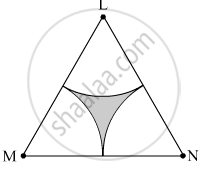SSC (Marathi Semi-English) 10thMaharashtra State Board
Share

# Δ Lmn is an Equilateral Triangle. Lm = 14 Cm. as Shown in Figure, Three Sectors Are Drawn with Vertices as Centres and Radius 7 Cm. Find, (1) a ( δ Lmn) - SSC (Marathi Semi-English) 10th - Geometry

ConceptAreas of Sector and Segment of a Circle

#### Question

$∆$ LMN is an equilateral triangle. LM = 14 cm. As shown in figure, three sectors are drawn with vertices as centres and radius 7 cm.
Find,
(1) A ( $∆$ LMN)#### Solution

∆LMN is an equilateral triangle.
∴ LM = MN = LN = 14 cm
∠L = ∠M = ∠N = 90º

(1)  Area of ∆LMN = $\frac{\sqrt{3}}{4} \left( \text{ Side} \right)^2 = \frac{\sqrt{3}}{4} \times \left( 14 \right)^2 = \frac{1 . 732}{4} \times 196$ =84.87 cm2

Is there an error in this question or solution?

#### APPEARS IN

Solution Δ Lmn is an Equilateral Triangle. Lm = 14 Cm. as Shown in Figure, Three Sectors Are Drawn with Vertices as Centres and Radius 7 Cm. Find, (1) a ( δ Lmn) Concept: Areas of Sector and Segment of a Circle.
S